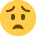# CSTR Sample Assessment 1 & 2

Hello,

Can anybody help me with task 7 on sample assessment 1 (question 8 on sample assessment 2)

I am really struggling to understand how to work out the margin of safety/margin of safety revenue and working it out as a percentageI have read over the answers over and over again but doesn't seem to help!

Thanks

• The margin of safety in units is the excess units after the break even point so in question 7 you have £14 variable costs per unit. £39.00 selling price means that after the variable costs (£14) you have a contribution per unit of £25.00. If fixed costs are £12 per unit based on 5000 the total fixed cost is £60000. so 60000/25 = 2400 BEP this is how many units you have to sell to cover the fixed costs.

The margin of safety is every unit produced after the BEP. so part C is 4000 units - BEP 2400= 1600.

the margin of safety as a % is : MOS in units/ forecasted 4000 units produced x100

1600/4000= .40 x100= 40%

part e) if a further 20k is added to the fixed costs of 60k the BEP in units is 80000/25= 3200BEP.
MOS in units is 5000- 3200= 1800
MOS % is 3200/ 5000 units produced x100 = 36%
MOS in revenue is 5000- 3200 = 1800 BEP units x £39.00 cost per item = 70200
• Thanks for your helpHappy to say I passed! now I only have 2 more exams to pass my level 3! yay